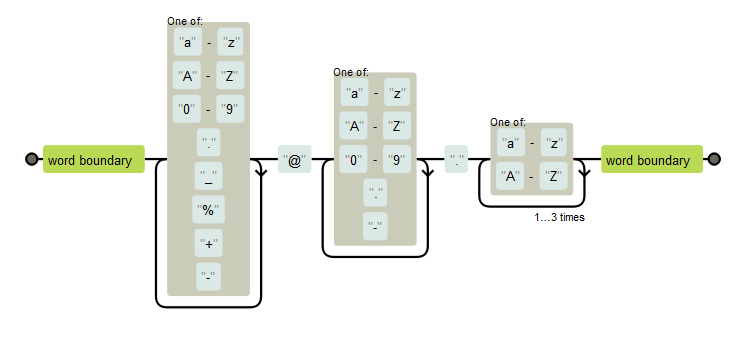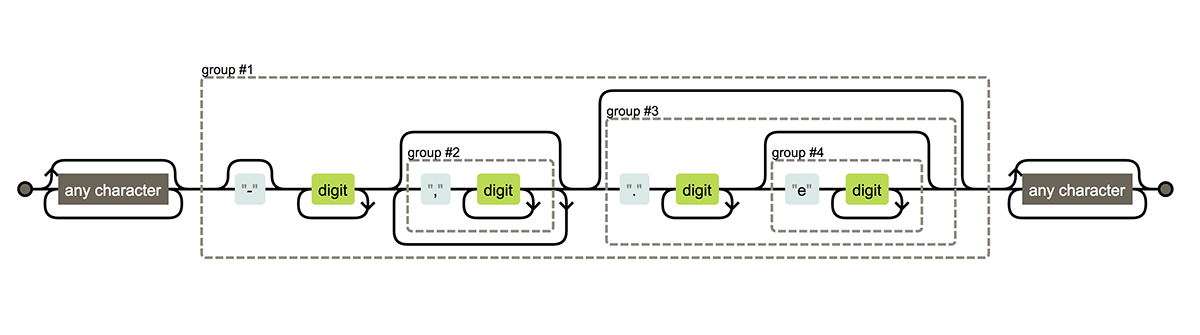/ re2r Public

RE2 Regular Expression in R.

# qinwf/re2r

Switch branches/tags
Nothing to show

A tag already exists with the provided branch name. Many Git commands accept both tag and branch names, so creating this branch may cause unexpected behavior. Are you sure you want to create this branch?

## Files

Failed to load latest commit information.
Type
Name
Commit time

# re2r

RE2 is a primarily DFA based regexp engine from Google that is very fast at matching large amounts of text.

## Installation

From CRAN:

`install.packages("re2r")`

From GitHub:

```library(devtools)
install_github("qinwf/re2r", build_vignettes = T)```

To learn how to use, you can check out the vignettes.

## Related Work

Google Summer of Code - re2 regular expressions.

## Brief Intro

### 1. Search a string for a pattern

`re2_detect(string, pattern)` searches the string expression for a pattern and returns boolean result.

```test_string = "this is just one test";
re2_detect(test_string, "(o.e)")```
``````##  TRUE
``````

Here is an example of email pattern.

`show_regex("\\b[a-zA-Z0-9._%+-]+@[a-zA-Z0-9.-]+\\.[a-zA-Z]{2,4}\\b", width = 670, height = 280)``re2_detect("test@gmail.com", "\\b[a-zA-Z0-9._%+-]+@[a-zA-Z0-9.-]+\\.[a-zA-Z]{2,4}\\b")`
``````##  TRUE
``````

`re2_match(string, pattern)` will return the capture groups in `()`.

`(res = re2_match(test_string, "(o.e)"))`
``````##      .match .1
## [1,] "one"  "one"
``````

The return result is a character matrix. `.1` is the first capture group and it is unnamed group.

Create named capture group with `(?P<name>pattern)` syntax.

`(res = re2_match(test_string, "(?P<testname>this)( is)"))`
``````##      .match    testname .2
## [1,] "this is" "this"   " is"
``````
`is.matrix(res)`
``````##  TRUE
``````
`is.character(res)`
``````##  TRUE
``````
`res\$testname`
``````## testname
##   "this"
``````

If there is no capture group, the matched origin strings will be returned.

```test_string = c("this is just one test", "the second test");
(res = re2_match(test_string, "is"))```
``````##      .match
## [1,] "is"
## [2,] NA
``````

`re2_match_all()` will return the all of patterns in a string instead of just the first one.

```res = re2_match_all(c("this is test",
"this is test, and this is not test",
"they are tests"),
pattern = "(?P<testname>this)( is)")
print(res)```
``````## []
##      .match    testname .2
## [1,] "this is" "this"   " is"
##
## []
##      .match    testname .2
## [1,] "this is" "this"   " is"
## [2,] "this is" "this"   " is"
##
## []
##      .match testname .2
``````
`is.list(res)`
``````##  TRUE
``````

match all numbers

```texts = c("pi is 3.14529..",
"-15.34 °F",
"128 days",
"1.9e10",
"123,340.00\$",
"only texts")
(number_pattern = re2(".*?(?P<number>-?\\d+(,\\d+)*(\\.\\d+(e\\d+)?)?).*?"))```
``````## re2 pre-compiled regular expression
##
## pattern: .*?(?P<number>-?\d+(,\d+)*(\.\d+(e\d+)?)?).*?
## number of capturing subpatterns: 4
## capturing names with indices:
## .match number .2 .3 .4
## expression size: 56
``````
`(res = re2_match(texts, number_pattern))`
``````##      .match          number       .2     .3       .4
## [1,] "pi is 3.14529" "3.14529"    NA     ".14529" NA
## [2,] "-15.34"        "-15.34"     NA     ".34"    NA
## [3,] "128"           "128"        NA     NA       NA
## [4,] "1.9e10"        "1.9e10"     NA     ".9e10"  "e10"
## [5,] "123,340.00"    "123,340.00" ",340" ".00"    NA
## [6,] NA              NA           NA     NA       NA
``````
`res\$number`
``````##  "3.14529"    "-15.34"     "128"        "1.9e10"     "123,340.00"
##  NA
``````
`show_regex(number_pattern)`### 2. Replace a substring

`re2_replace(string, pattern, rewrite)`

Searches the string "input string" for the occurence(s) of a substring that matches 'pattern' and replaces the found substrings with "rewrite text".

```input_string = "this is just one test";
new_string = "my"
re2_replace(new_string, "(o.e)", input_string)```
``````##  "my"
``````

mask the middle three digits of a US phone number

```texts = c("415-555-1234",
"650-555-2345",
"(416)555-3456",
"202 555 4567",
"4035555678",
"1 416 555 9292")

us_phone_pattern = re2("(1?[\\s-]?\\(?\\d{3}\\)?[\\s-]?)(\\d{3})([\\s-]?\\d{4})")

re2_replace(texts, us_phone_pattern, "\\1***\\3")```
``````##  "415-***-1234"   "650-***-2345"   "(416)***-3456"  "202 *** 4567"
##  "403***5678"     "1 416 *** 9292"
``````

### 3. Extract a substring

`re2_extract(string, pattern, replacement)`

Extract matching patterns from a string.

`re2_extract("yabba dabba doo", "(.)")`
``````##  "y"
``````
`re2_extract("test@me.com", "(.*)@([^.]*)")`
``````##  "test@me"
``````

### 4. `Regular Expression Object` for better performance

We can create a regular expression object (RE2 object) from a string. It will reduce the time to parse the syntax of the same pattern.

And this will also give us more option for the pattern. run `help(re2)` to get more detials.

```regexp = re2("test",case_sensitive = FALSE)
print(regexp)```
``````## re2 pre-compiled regular expression
##
## pattern: test
## number of capturing subpatterns: 0
## capturing names with indices:
## .match
## expression size: 10
``````
```regexp = re2("test",case_sensitive = FALSE)
re2_match("TEST", regexp)```
``````##      .match
## [1,] "TEST"
``````
`re2_replace("TEST", regexp, "ops")`
``````##  "ops"
``````

Use `parallel` option to enable multithread feature. It will improve performance for large inputs with a multi core CPU.

`re2_match(string, pattern, parallel = T)`

RE2 Regular Expression in R.

## Releases

No releases published

## Packages 0

No packages published